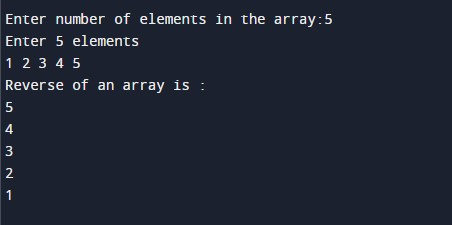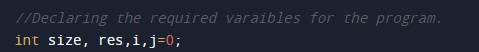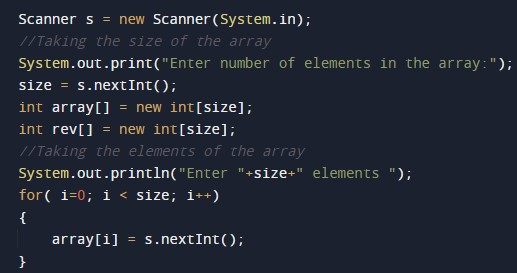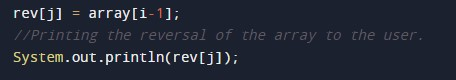# Java Program to reverse an array

In this tutorial you will learn about the Java Program to reverse an array and its application with practical example.

## Java Program to Reverse an Array.

In this tutorial, we will learn to create a Java program that will reverse an Array Using Java programming.

## Prerequisites

Before starting with this tutorial, we assume that you are the best aware of the following Java programming topics:

• Operators in Java Programming.
• Basic Input and Output function in Java Programming.
• Basic Java programming.
• For loop in Java programming.
• Arithmetic operations in Java Programming.

## Reversing an Array:-

In this program, First, we will first take the size of the array from the user. Then we will take the elements of the array from the user. Then we will copy elements of the array. After that, we will reverse the array using the for-loop. Then we will print the reverse of the original array to the user.

With the help of this program, we can be reverse an Array.

## Output:-In the above program, we have first initialized the required variable.• array[] = it will hold the integer value.
• i = it will hold the integer value.
• j = it will hold the integer value.
• size = it will hold the integer value.

Taking the size of the array and the elements.Program Code to Reverse an Array.Printing reversed array to the user.In this tutorial we have learn about the Java Program to reverse an array and its application with practical example. I hope you will like this tutorial.